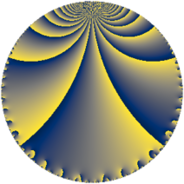# Properties

 Label 112.4.aLevel $112$ Weight $4$ Character orbit 112.a Rep. character $\chi_{112}(1,\cdot)$ Character field $\Q$ Dimension $9$ Newform subspaces $8$ Sturm bound $64$ Trace bound $5$

# Related objects

## Defining parameters

 Level: $$N$$ $$=$$ $$112 = 2^{4} \cdot 7$$ Weight: $$k$$ $$=$$ $$4$$ Character orbit: $$[\chi]$$ $$=$$ 112.a (trivial) Character field: $$\Q$$ Newform subspaces: $$8$$ Sturm bound: $$64$$ Trace bound: $$5$$ Distinguishing $$T_p$$: $$3$$, $$5$$

## Dimensions

The following table gives the dimensions of various subspaces of $$M_{4}(\Gamma_0(112))$$.

Total New Old
Modular forms 54 9 45
Cusp forms 42 9 33
Eisenstein series 12 0 12

The following table gives the dimensions of the cuspidal new subspaces with specified eigenvalues for the Atkin-Lehner operators and the Fricke involution.

$$2$$$$7$$FrickeDim.
$$+$$$$+$$$$+$$$$2$$
$$+$$$$-$$$$-$$$$2$$
$$-$$$$+$$$$-$$$$2$$
$$-$$$$-$$$$+$$$$3$$
Plus space$$+$$$$5$$
Minus space$$-$$$$4$$

## Trace form

 $$9q + 2q^{5} + 7q^{7} + 101q^{9} + O(q^{10})$$ $$9q + 2q^{5} + 7q^{7} + 101q^{9} - 76q^{11} - 46q^{13} + 72q^{15} - 102q^{17} + 24q^{19} + 56q^{23} + 247q^{25} + 576q^{27} - 242q^{29} - 264q^{31} - 384q^{33} - 210q^{35} + 326q^{37} - 264q^{39} + 98q^{41} - 28q^{43} + 90q^{45} + 792q^{47} + 441q^{49} - 1200q^{51} - 98q^{53} - 1080q^{55} + 40q^{57} - 960q^{59} - 1302q^{61} + 315q^{63} - 116q^{65} + 36q^{67} - 528q^{69} + 840q^{71} + 458q^{73} + 2720q^{75} + 476q^{77} + 304q^{79} + 1577q^{81} + 1112q^{83} - 700q^{85} - 16q^{87} - 150q^{89} - 546q^{91} - 1680q^{93} + 1192q^{95} + 906q^{97} + 2308q^{99} + O(q^{100})$$

## Decomposition of $$S_{4}^{\mathrm{new}}(\Gamma_0(112))$$ into newform subspaces

Label Dim. $$A$$ Field CM Traces A-L signs $q$-expansion
$$a_2$$ $$a_3$$ $$a_5$$ $$a_7$$ 2 7
112.4.a.a $$1$$ $$6.608$$ $$\Q$$ None $$0$$ $$-8$$ $$-14$$ $$7$$ $$-$$ $$-$$ $$q-8q^{3}-14q^{5}+7q^{7}+37q^{9}+28q^{11}+\cdots$$
112.4.a.b $$1$$ $$6.608$$ $$\Q$$ None $$0$$ $$-6$$ $$8$$ $$7$$ $$+$$ $$-$$ $$q-6q^{3}+8q^{5}+7q^{7}+9q^{9}-56q^{11}+\cdots$$
112.4.a.c $$1$$ $$6.608$$ $$\Q$$ None $$0$$ $$-4$$ $$6$$ $$-7$$ $$-$$ $$+$$ $$q-4q^{3}+6q^{5}-7q^{7}-11q^{9}+12q^{11}+\cdots$$
112.4.a.d $$1$$ $$6.608$$ $$\Q$$ None $$0$$ $$2$$ $$-16$$ $$7$$ $$+$$ $$-$$ $$q+2q^{3}-2^{4}q^{5}+7q^{7}-23q^{9}-24q^{11}+\cdots$$
112.4.a.e $$1$$ $$6.608$$ $$\Q$$ None $$0$$ $$2$$ $$-12$$ $$-7$$ $$-$$ $$+$$ $$q+2q^{3}-12q^{5}-7q^{7}-23q^{9}-48q^{11}+\cdots$$
112.4.a.f $$1$$ $$6.608$$ $$\Q$$ None $$0$$ $$2$$ $$16$$ $$7$$ $$-$$ $$-$$ $$q+2q^{3}+2^{4}q^{5}+7q^{7}-23q^{9}+8q^{11}+\cdots$$
112.4.a.g $$1$$ $$6.608$$ $$\Q$$ None $$0$$ $$10$$ $$-8$$ $$7$$ $$-$$ $$-$$ $$q+10q^{3}-8q^{5}+7q^{7}+73q^{9}+40q^{11}+\cdots$$
112.4.a.h $$2$$ $$6.608$$ $$\Q(\sqrt{57})$$ None $$0$$ $$2$$ $$22$$ $$-14$$ $$+$$ $$+$$ $$q+(1+\beta )q^{3}+(11+\beta )q^{5}-7q^{7}+(31+\cdots)q^{9}+\cdots$$

## Decomposition of $$S_{4}^{\mathrm{old}}(\Gamma_0(112))$$ into lower level spaces

$$S_{4}^{\mathrm{old}}(\Gamma_0(112)) \cong$$ $$S_{4}^{\mathrm{new}}(\Gamma_0(7))$$$$^{\oplus 5}$$$$\oplus$$$$S_{4}^{\mathrm{new}}(\Gamma_0(8))$$$$^{\oplus 4}$$$$\oplus$$$$S_{4}^{\mathrm{new}}(\Gamma_0(14))$$$$^{\oplus 4}$$$$\oplus$$$$S_{4}^{\mathrm{new}}(\Gamma_0(16))$$$$^{\oplus 2}$$$$\oplus$$$$S_{4}^{\mathrm{new}}(\Gamma_0(28))$$$$^{\oplus 3}$$$$\oplus$$$$S_{4}^{\mathrm{new}}(\Gamma_0(56))$$$$^{\oplus 2}$$